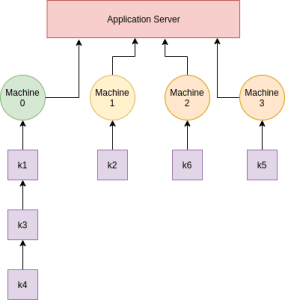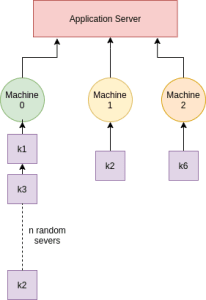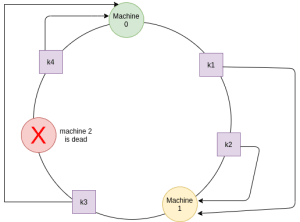Home System Design Tutorial Consistent Hashing - System Design

# Consistent Hashing – System Design

Consistent hashing is done to implement scalability into the storage system by dividing up the data among multiple storage servers.

We use consistent hashing when we have lots of data distributed among lots of servers(database server), and the number of available servers changes continuously(either a new server added or a server is removed).

### Why not Simple Hashing

A simple hash function will take the data/key and produce a number in a specified range.

Suppose that we have large chunks of data, we are first calculating a hash of it(say using md5). This is done so that we can get a random value in a range [0 – 2^(128)

Now, our hash function will  look something like this
server_number = hash(key) % n, this will give a random number in the range [0-(n-1)] and n is the number of servers.

This will divide the data perfectly among the number of servers.

Example -> number of servers, n = 3 and we have 6 keys
keys          hash value     server_number(hashing)
key1            100                1
key2            577                1
key3            872                2
key4            376                0
key5            23                 2
key6            6798               0

This is how the keys will be distributed among 3 servers:

The problem arises when a server is added.

We need to add the keys to this new server too, and for this, we have to recompute all hash again(with numbers servers=4) to find the server number for each key and hence the key distribution will change.

Example -> number of servers, n = 4 and we have 6 keys
keys          hash value     server_number(hashing)
key1            100                0
key2            577                1
key3            872                0
key4            376                0
key5            23                 3
key6            6798               2New server, server 3 is added, hence all keys need to be rehashed. Mapping of keys to various database servers using simple hashing

The same kind of problem arises when a server is removed, we have to recompute hash(with a new number of servers).

Another problem that we will encounter is skewing i.e, too many keys will hash to one server and too little to others or keys are non-uniformly distributed.

######The major problems we face with simple/regular hashing:
1. Adding a server/server to the ring.
2. Removing a server/server to the ring.
3. Non-Uniform distribution of keys across the ring.

## Consistent Hashing

Consistent hashing helps us to distribute data across a set of nodes/servers in such a way that reorganization is minimum. The magic of consistent hashing lies in the way we are assigning keys to the servers.

In-consistent hashing, the hash function works independently of the number of nodes/servers. Here we assume a virtual ring is formed and keys, servers are distributed around the ring.

The hash function is   position = hash(key) % (2^32 ). Here 2^32 is the number of positions (or ring length) and is a completely random number, you can choose any large number of your choice.

###### Finding the position for the server(database server) and the keys in the ring
1. To find the position of the server, we can take the hash of the Ip address of the server and then calculate its position using the above hash function.
2. Find the hash of each key and find its position using the above hash function and place it at that particular position.
3. Place the server at the calculated positions in the ring.
4. Map keys to the server, which has the same hash value as that of the key( key hash value == server hash value).
5. In case the key hash value doesn’t match any server, the key will be mapped to the nearest server in the clockwise direction.

###### Adding a new server to the ring

Let’s say we are going to add a new server and says its hash value lies between server 0 and server 1.

As a result of adding a new server, we don’t have to rehash all the key values, instead only those which lie in between server 0 and server 1. On average in real-time, if we have n servers and k keys, we have to rehash only k/n keys. This is a significant improvement over the simple hashing.

###### Removing a server from the ring

In case a server goes down then we have to rehash only keys stored in it and the keys stored in its clockwise neighboring server. Suppose server 2 goes down.Server 2 is dead and all of its corresponding keys only are rehashed. Consistent Hashing – server is removed

###### Non-Uniform Distribution

There can be one more problem that can appear. The non-uniform hashing problem i.e, the majority of keys get hashed to a single machine or near to the single server. This is not ideal as one server has more number of keys as compared to all other servers.